# These 20 pandas functions can improve your 'data cleaning' ability by 100 times

2022-02-02 07:40:58 jiejie

Hello everyone , I am a Jiejie

Today I'm going to introduce an article ` Super liver goods `！**Pandas ** Is based on NumPy A tool of , The tool was created to solve the task of data analysis . It provides a large number of functions and methods that enable us to process data quickly and easily . This article introduces 20 individual 【 Divided into 15 Group 】 function , Is absolutely ` Data processing ` killer , You'll love it when you use it .## Construct data set

Let's start here ` Construct a dataset `, Used to demonstrate this 20 A function .

``````import pandas as pd
df ={' full name ':['  Schoolmate Huang ',' Huang Zhizun ',' Huanglaoxie  ',' Chen Dami ',' Sun shangxiang '],
' English name ':['Huang tong_xue','huang zhi_zun','Huang Lao_xie','Chen Da_mei','sun shang_xiang'],
' Gender ':[' male ','women','men',' Woman ',' male '],
' Id card ':['463895200003128433','429475199912122345','420934199110102311','431085200005230122','420953199509082345'],
' Home address ':[' Guangshui, Hubei Province ',' Xinyang, Henan ',' Guangxi Guilin ',' Xiaogan, Hubei ',' Guangdong guangzhou '],
' Phone number ':['13434813546','19748672895','16728613064','14561586431','19384683910'],
' income ':['1.1 ten thousand ','8.5 thousand ','0.9 ten thousand ','6.5 thousand ','2.0 ten thousand ']}
df = pd.DataFrame(df)
df
Copy code ``````

design sketch ：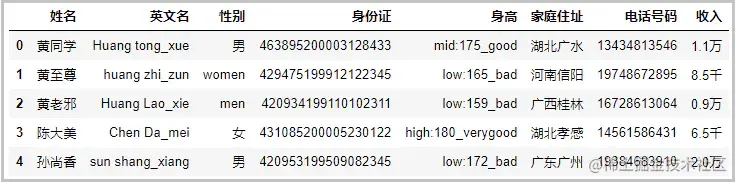## 1. cat function

This function is mainly used for ` String splicing `;

``````df[" full name "].str.cat(df[" Home address "],sep='-'*3)
Copy code ``````

design sketch ：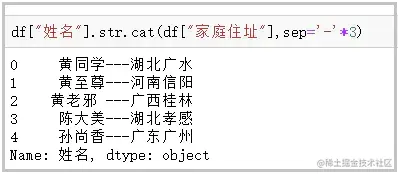## 2. contains function

This function is mainly used for ` Determine whether a string contains a given character `;

``````df[" Home address "].str.contains(" wide ")</pre>
Copy code ``````

design sketch ：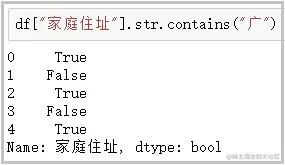## 3. startswith、endswith function

This function is mainly used for ` Determine whether a string is represented by ... start / ending `;

``````#  The first line “  Huang Wei ” It starts with a space
df[" full name "].str.startswith(" yellow ")
df[" English name "].str.endswith("e")</pre>
Copy code ``````

design sketch ：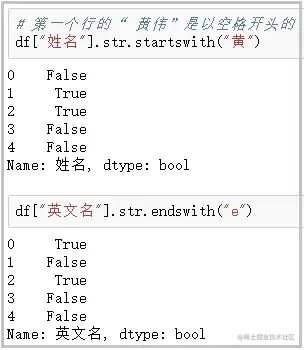## 4. count function

This function is mainly used for ` Calculates the number of occurrences of a given character in a string `;

``````df[" Phone number "].str.count("3")</pre>
Copy code ``````

design sketch ：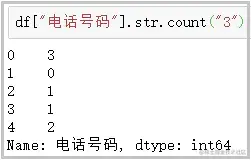## 5. get function

This function is mainly used for ` Gets the string at the specified location `;

``````df[" full name "].str.get(-1)
df[" height "].str.split(":")
df[" height "].str.split(":").str.get(0)</pre>
Copy code ``````

design sketch ：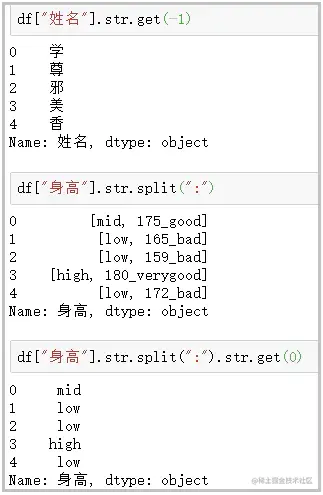## 6. len function

This function is mainly used for ` Calculate string length `;

``````df[" Gender "].str.len()</pre>
Copy code ``````

design sketch ：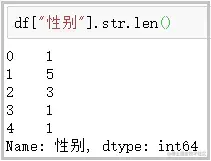## 7. upper、lower function

This function is mainly used for ` English case conversion `;

``````df[" English name "].str.upper()
df[" English name "].str.lower()</pre>
Copy code ``````

design sketch ：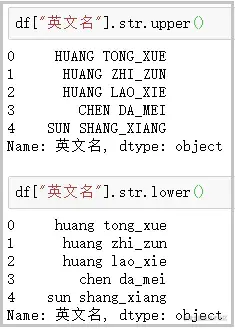## 8. pad+side Parameters /center function

This function is mainly used for ` To the left of the string 、 Add the given character to the right or left `;

``````df[" Home address "].str.pad(10,fillchar="*")      #  amount to ljust()
df[" Home address "].str.pad(10,side="right",fillchar="*")    #  amount to rjust()
df[" Home address "].str.center(10,fillchar="*")</pre>
Copy code ``````

design sketch ：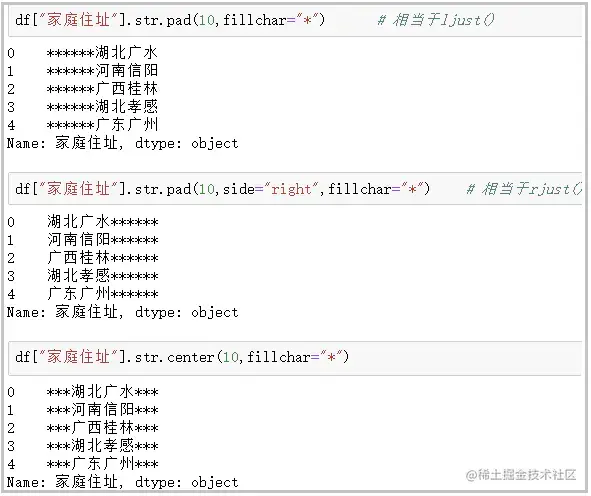## 9.  repeat function

This function is mainly used for ` Repeat string several times `;

``````df[" Gender "].str.repeat(3)</pre>
Copy code ``````

design sketch ：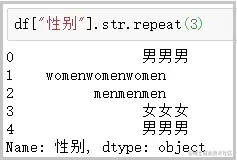## 10.  slice_replace function

This function is mainly used for ` Use the given string , Replace the character at the specified position `;

``````df[" Phone number "].str.slice_replace(4,8,"*"*4)</pre>
Copy code ``````

design sketch ：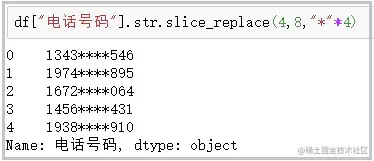## 11. replace function

This function is mainly used for ` The character at the specified position , Replace with the given string `;

``````df[" height "].str.replace(":","-")</pre>
Copy code ``````

design sketch ：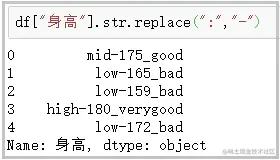This function also ` Accept regular expressions `, The character at the specified position , Replace with the given string .

``````df[" income "].str.replace("\d+\.\d+"," Regular ")</pre>
Copy code ``````

design sketch ：## 12.  split Method +expand Parameters

This function is mainly used for ` Expand a column into several columns `;

``````#  Common usage
df[" height "].str.split(":")
# split Method , collocation expand Parameters
df[[" Height description ","final height "]] = df[" height "].str.split(":",expand=True)
df
# split Method collocation join Method
df[" height "].str.split(":").str.join("?"*5)</pre>
Copy code ``````

design sketch ：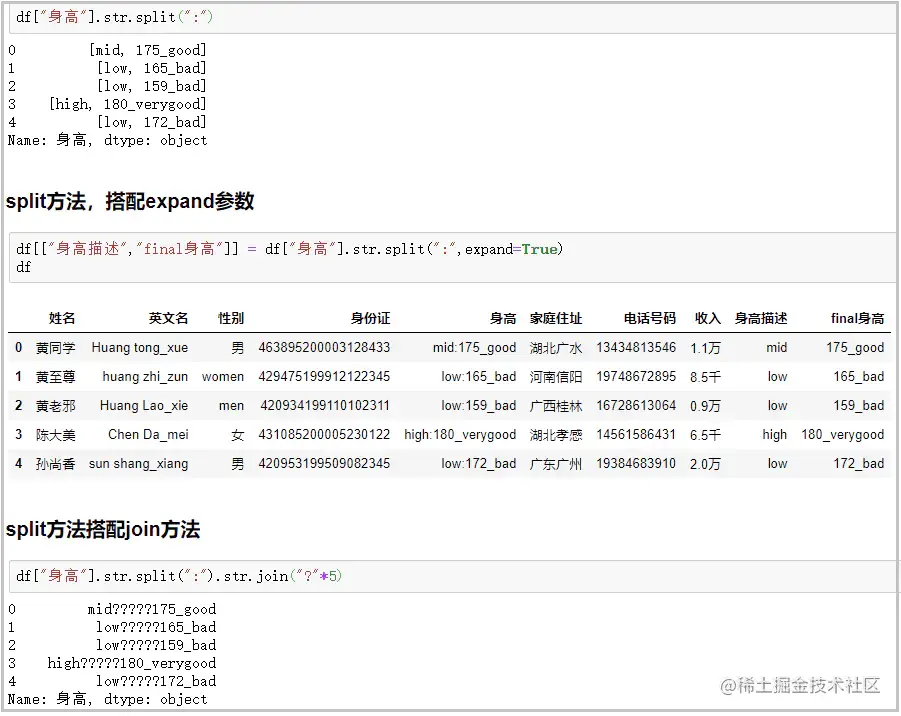## 13. strip、rstrip、lstrip function

This function is mainly used for ` Remove blanks 、 A newline `;

``````df[" full name "].str.len()
df[" full name "] = df[" full name "].str.strip()
df[" full name "].str.len()</pre>
Copy code ``````

design sketch ：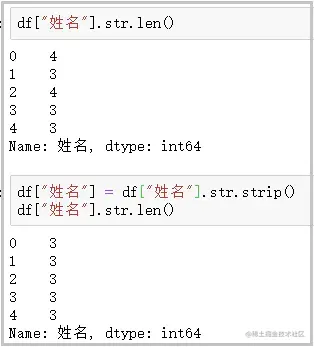## 14. findall function

This function is mainly used for ` Using regular expressions , To match... In a string , Returns a list of search results `;

``````df[" height "]
df[" height "].str.findall("[a-zA-Z]+")</pre>
Copy code ``````

design sketch ：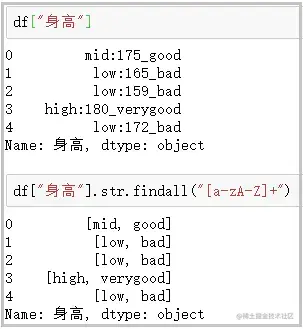## 15. extract、extractall function

This function is mainly used for ` Accept regular expressions , Extract the matching string ( Be sure to put parentheses )`;

``````df[" height "].str.extract("([a-zA-Z]+)")
# extractall Extract the composite index
df[" height "].str.extractall("([a-zA-Z]+)")
# extract collocation expand Parameters
df[" height "].str.extract("([a-zA-Z]+).*?([a-zA-Z]+)",expand=True)</pre>
Copy code ``````

design sketch ：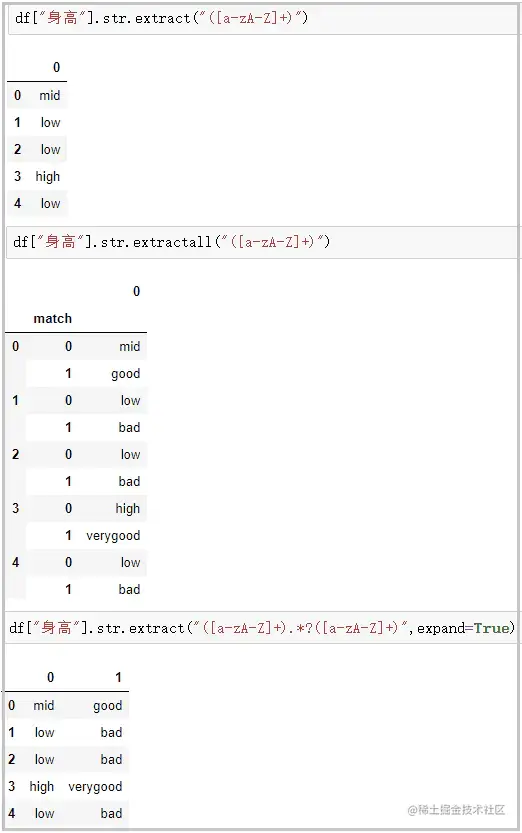If you think this article , If it's of some use to you , Don't forget the third company , Because this will be the strongest driving force for me to continue to output more quality articles ！